# Getting started with finaquant® protos 1.0

Four simple examples related with price calculation and cost aggregation are presented below to give you some feeling about table functions. These examples are included in the demonstration function `Getting_started_with_finaquant_protos` in the code file Demo.cs in Visual Studio project FinaquantProtosStarter. You just need to run this demo function by pressing F5 after placing the cursor in a code file.

Increase video quality to 480p if you don’t see the pictures clearly

### Define fields shared by all tables centrally in MetaData

```// define table structure var CostTableFields = TableFields.CreateEmptyTableFields(md); TableFields.AddNewField(CostTableFields, "category"); TableFields.AddNewField(CostTableFields, "product"); TableFields.AddNewField(CostTableFields, "year"); TableFields.AddNewField(CostTableFields, "costs"); // create cost table with elements MatrixTable CostTable = MatrixTable.CreateTableWithElements_A(CostTableFields, "Computer", "HP", 2008, 1200.0, "Computer", "Toshiba", 2008, 800.0, "Computer", "Asus", 2010, 900.0, "Computer", "Asus", 2011, 1100.0, "Computer", "HP", 2010, 750.0, "Computer", "Toshiba", 2010, 950.0, "Mobile phone", "Nokia", 2008, 300.0, "Mobile phone", "Motorola", 2008, 250.0, "Mobile phone", "Nokia", 2010, 200.0, "Mobile phone", "Motorola", 2010, 150.0 ); // view CostTable MatrixTable.View_MatrixTable(CostTable, "Cost table")```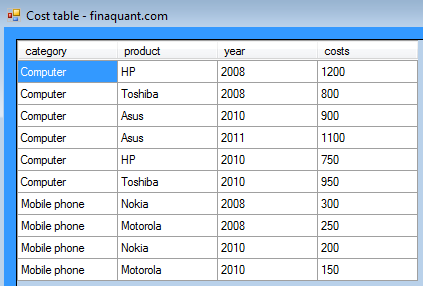### Example 1: Calculate prices; price margins are specified per category

Related help page: Table arithmetics
General formula: price = costs x (1 + margin)

```// create the first price margin table var MarginTableFields1 = TableFields.CreateEmptyTableFields(md); TableFields.AddNewField(MarginTableFields1, "category"); TableFields.AddNewField(MarginTableFields1, "margin"); // margins per category MatrixTable MarginTable1 = MatrixTable.CreateTableWithElements_A(MarginTableFields1, "Computer", 0.25, "Mobile phone", 0.40); // view MarginTable1 MatrixTable.View_MatrixTable(MarginTable1, "Margins per category")```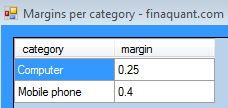```// add 1 to margins MatrixTable MarginTable1x = MatrixTable.AddScalarToAllKeyFigures(MarginTable1, 1.0); // price = cost x (1 + margin) MatrixTable PriceTable1 = MatrixTable.MultiplySelectedKeyFigures( CostTable, MarginTable1x, InputKeyFigTbl1: "costs", InputKeyFigTbl2: "margin", OutputKeyFig: "price", MultiplyRestWith: 1.0); // view PriceTable1 MatrixTable.View_MatrixTable(PriceTable1, "First price table, calculated with margins per category")```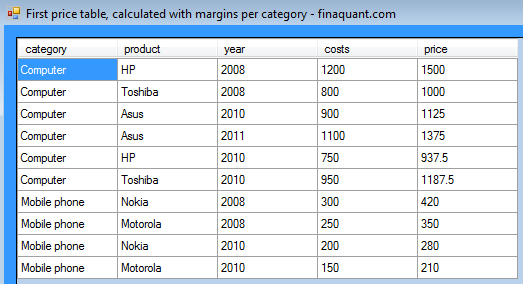Note that resultant price margins are 25% for computers, and 40% for mobile phones, as seen in the table above.

### Example 2: Calculate prices; price margins are specified per product and year

In this example, price margins are specified in more detail; not per category this time, but per product and year. The default price margin is 20% if the margin for a product-year pair is not specified in the margin table.

```// create the second price margin table var MarginTableFields2 = TableFields.CreateEmptyTableFields(md); TableFields.AddNewField(MarginTableFields2, "product"); TableFields.AddNewField(MarginTableFields2, "year"); TableFields.AddNewField(MarginTableFields2, "margin"); // margins specified per product and year MatrixTable MarginTable2 = MatrixTable.CreateTableWithElements_A(MarginTableFields2, "HP", 2008, 0.25, "HP", 2010, 0.35, "Asus", 2010, 0.30, "Toshiba", 2008, 0.25, "Nokia", 2008, 0.45, "Motorola", 2008, 0.40 ); // view MarginTable2 MatrixTable.View_MatrixTable(MarginTable2, "Margins per product and year")```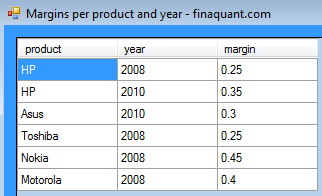```// add 1 to margins MatrixTable MarginTable2x = MatrixTable.AddScalarToAllKeyFigures(MarginTable2, 1.0); // price = cost x (1 + margin) // default margin for unspecified product-year pairs: 1.20 MatrixTable PriceTable2 = MatrixTable.MultiplySelectedKeyFigures(CostTable, MarginTable2x, InputKeyFigTbl1: "costs", InputKeyFigTbl2: "margin", OutputKeyFig: "price", MultiplyRestWith: 1.20 ); // view PriceTable2 MatrixTable.View_MatrixTable(PriceTable1, "Second price table, calculated with margins per product and year")```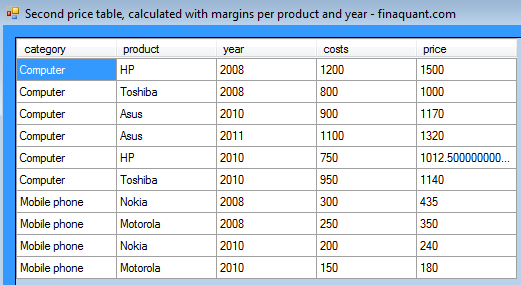Note that a price margin of 20% was applied on the product-year pair Toshiba-2010, because its margin was not explicitly specified.

As specified in the margin table, a price margin of 25% was applied on HP-2008, and 35% on HP-2010.

### Example 3: Aggregate cost table to obtain costs per category

Related help page: Table aggregation functions

In this example, a summary table with two fields (costs, category) is derived by aggregating the initial cost table. This aggregated table shows total costs per category. That is, the aggregation function is SUM. As shown below, the desired aggregation is accomplished in two steps:

```// partition cost table to obtain costs per category before aggregation MatrixTable CostsPerCategory = MatrixTable.PartitionColumn(CostTable, TextVector.CreateVectorWithElements("category", "costs")); // view CostsPerCategory before aggregation MatrixTable.View_MatrixTable(CostsPerCategory, "Costs per category, before aggregation of costs")```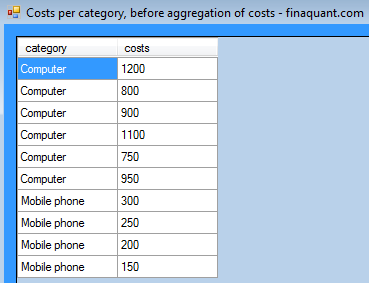```// aggregate costs with default aggregation function SUM CostsPerCategory = MatrixTable.AggregateAllKeyFigures(CostsPerCategory, null); // view CostsPerCategory after aggregation MatrixTable.View_MatrixTable(CostsPerCategory, "Total costs per category, after aggregation of costs")```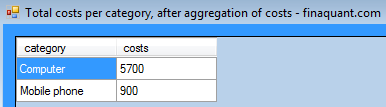### Example 4: Add new key figure “total costs per category” into cost table

In this example, the initial cost table is not aggregated to obtain the summary table (costs per category), but a new key figure `total_costs_category` is added into the table as additional summary information. For finaquant® protos this is just another kind of aggregation.

```bool ifsuccess; string WarningMsg; // Aggregate selected key figure MatrixTable CostTableAdj = MatrixTable.AggregateSelectedKeyFigure_B(CostTable, RefAttributes: TextVector.CreateVectorWithElements("category"), InputKeyFigName: "costs", OutputKeyFigName: "total_costs_category", AggrOpt: AggregateOption.nSum, IfSuccess: out ifsuccess, Warnings: out WarningMsg); // view CostTableAdj MatrixTable.View_MatrixTable(CostTableAdj, "Cost table with added key figure total costs per category")```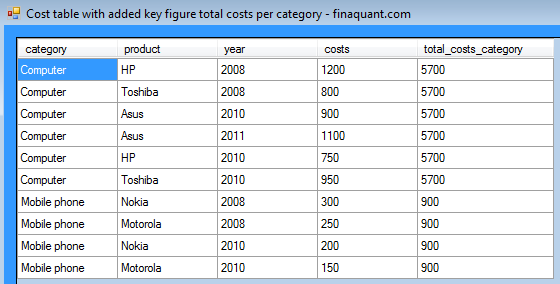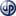Copyright secured by Digiprove © 2012 Tunc Ali Kütükcüoglu
This entry was posted in Calculation engine and tagged , , . Bookmark the permalink.

### 2 Responses to Getting started with finaquant® protos 1.0

1.ugurtanriverdi says:

Hi Tunc

Quite impressive work thanks for sharing.

If i understood correctly, with your new library, you are bringing a new alternative to development environments like (SQL-DB), (matlab-matrix operations) so this peace of code, only needs Visual Studio and your library to manage such operations, like join, aggregation etc…

If so, apart having possibility to use table/matrix/array level operations without any dependency to specific DB’s (oracle, SQLServer, DB2 etc…) or tools/environments (R, Mathlab etc…), your library brings any further concrete advantages, to consider it much more convenient than it’s alternatives or you mainly you tried to bring a compact, chip replacement, to these relatively difficult to maintain and expensive commercial solutions?

Thanks & Regards

•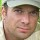tuncalik says:

Hi Ugur,

Yes, table calculations directly within the .NET framework, with in-memory data is one aspect of our calculation engines. These table functions are the infrastructure of an upcoming real calculation engine based on table functions.

The other aspect will be features like ability to define and maintain network calculations with multiple chained nodes (or contracts), simplified parameter management etc. that will be built on this infrastructure. Only these additional features will make our products real calculation engines.

I am also a proficient user of math software like matlab or R. There are cases where you want to make operations with table data. Converting these tables first to matrices, making the operations, and then everything back to tables is an error-prone and tedious task. Table functions offer a more direct and intuitive way of doing operations on tables. Once (and if) you get used to working with tables as a matrix guru, you will understand what I mean; you won’t want to go back to matrices for structured higher-level table data unless it is strictly necessary.

I haven’t seen so far a software (maybe there are some, but I coudn’t find any) including stored procedures of certain databases, which offers table functions in the entirety and with the mathematical clarity as finaquant intends to do. I have seen some patches table functions embedded in expensive software for applications like financial planning and reporting, but they are not extensive, complete, and well-defined (in the mathematical sense) enough for users with some analytical flair, who want to configure (or program) their calculations themselves without much reliance on external support.

Regards, Tunc